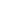Flat 50% off

Ends in

• NEET
•• 12th
•• Physics
• Back to Doubt Clearing

## Answer given questionTwo identical conducting spheres carrying different charges attract each other with a force $F$ when placed in air medium at a distance $d$ apart. The sphere are brought into contact and then taken to their original positions. Now, the two spheres repel each other with a force whole magnitude is equal to that of the initial attractive force. The ratio between initial charges on the spheres is(a) $-(3 + \sqrt 8)$ only(b) $-3 + \sqrt 8$ only(c) $-(3 + \sqrt 8)$ or $(-3 + \sqrt 8)$ (d) $+ \sqrt 3$(e) $- \sqrt 8$

### Asked By Nikhil kursunge

Updated Wed, 21 Nov 2018 03:12 pm